Concept 5: Measures of Central Tendency | IFT World
101 Concepts for the Level I Exam

# Concept 5: Measures of Central TendencyArithmetic mean is the sum of all the observations divided by the total number of observations. A population average is called population mean (µ). A sample average is called sample mean (). The sample mean is used as the ‘best guess’ approximation of the population mean.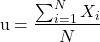A stock had the following returns in the past three years: 10%, -5%, and 20%. Calculate the arithmetic mean.

Solution:

Arithmetic mean = (10 – 5 + 20)/3 = 8.33%

Median is the midpoint of a data set that has been sorted from largest to smallest. If we have an even number of observations, then the median is the average of the two middle observations.

Calculate the medians for the following data sets:

1. 1, 2, 3, 4, 5
2. 1, 2, 3, 4, 5, 6

Solution:

1. Median = 3
2. Median = (3+4)/2 = 3.5

Mode is the value that occurs most frequently in a data set. A data set can have more than one mode, but only one mean and one median.

Calculate the mode for the following data set: 1, 2, 2, 3, 4, 4, 4, 5, 5

Solution:

Mode = 4

Geometric mean is used to calculate compound growth rate.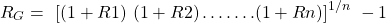A stock had the following returns in the past three years: 5%, -5%, and 20%. Calculate the geometric mean.

Solution: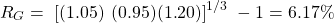In a weighted mean different observations are given different weights as per their proportional influence on the mean.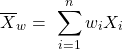An investor has 20% of his portfolio in Stock A, 30% in Stock B and 50% in Stock C. If the returns were 4% on Stock A, 7% on Stock B and 8% on Stock C. Calculate portfolio return.

Solution:

Portfolio return = 0.2 x 4 + 0.3 x 7 + 0.5 x 8 = 6.9%

Harmonic mean is used to find average purchase price for equal periodic investments.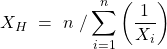An investor purchased $1,000 worth of stock A each month for the past three months at prices of$5, $6 and$7. Calculate the average purchase price of the stock.

Solution:

Average purchase price = 3 / (1/5 + 1/6 + 1/7) = 5.88

If returns are constant over time: AM = GM = HM

If returns are variable over time: AM > GM > HM

Crash Courses for November CFA Level I, II exams are coming soon!
This is default text for notification bar